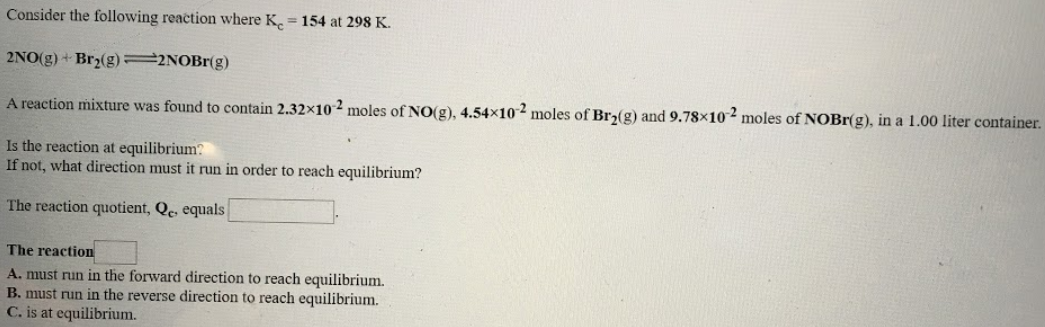# Consider the following reaction where Kc = 154 at 298 K. 2NO(g) + Br2(g) ⇌ 2NOBr2(g) A reaction mixture was found to contain 2.32x10^-2 moles of NO(g), 4.54x10^-2 moles of Br2(g) and 9.78x10^-2 moles of NOBr(g), in a 1.00 liter container. Is the reaction at equilibrium? If not, what direction must it run in order to reach equilibrium? The reaction quotient, Qc, equals ____. The reaction ____. A. must run in the forward direction to reach equilibrium. B. must run in the reverse direction to reach equilibrium. C. is at equilibrium.